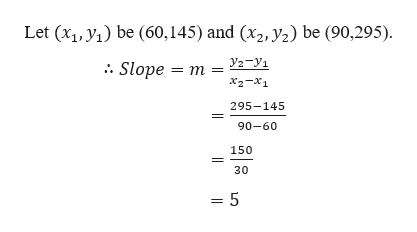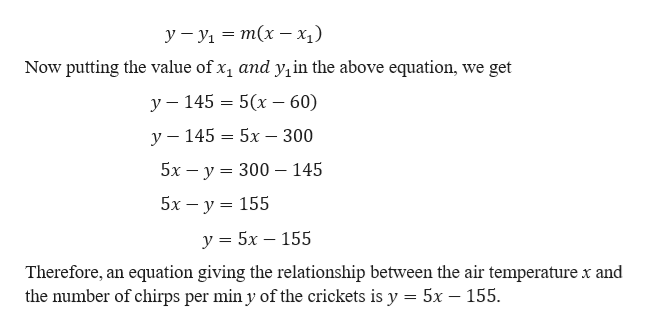# ntomologists have discovered that a linear relationship exists between the rate of chirping of crickets of a certain species and the air temperature. When the temperature is 60°F, the crickets chirp at the rate of 145 chirps/min, and when the temperature is 90°F, they chirp at the rate of 295 chirps/min.(a) Find an equation giving the relationship between the air temperature x and the number of chirps per min y of the crickets.(b) Use this function to determine the rate at which the crickets chirp when the temperature is 101°F.chirps/min

Question
18 views

ntomologists have discovered that a linear relationship exists between the rate of chirping of crickets of a certain species and the air temperature. When the temperature is 60°F, the crickets chirp at the rate of 145 chirps/min, and when the temperature is 90°F, they chirp at the rate of 295 chirps/min.

(a) Find an equation giving the relationship between the air temperature x and the number of chirps per min y of the crickets.

(b) Use this function to determine the rate at which the crickets chirp when the temperature is 101°F.
chirps/min
check_circle

Step 1

Part A

When the temperature is 60°F, the crickets chirp at the rate of 145 chirps/min, and when the temperature is 90°F, they chirp at the rate of 295 chirps/min.

Let x represents the air temperature and y represents chirps rate.help_outlineImage TranscriptioncloseLet (x1, y) be (60,145) and (x2, y2) be (90,295) y2-y1 Slope m x2-x1 295-145 90-60 150 30 5 Ln fullscreen
Step 2

Finding the equation using the slope-point form i.e.help_outlineImage Transcriptioncloseу — У1 — т(х — х) Now putting the value of x1 and yin the above equation, get we у — 145 — 5(х — 60) — 300 у — 145 3D 5х 5х — у %3D 300 — 145 5х — у — 155 у %3 5х — 155 Therefore, an equation giving the relationship between the air temperature x and the number of chirps per min y of the crickets is y = 5x - 155 fullscreen
Step 3

Part b

...

### Want to see the full answer?

See Solution

#### Want to see this answer and more?

Solutions are written by subject experts who are available 24/7. Questions are typically answered within 1 hour.*

See Solution
*Response times may vary by subject and question.
Tagged in

### Equations and In-equations